# 机器学习A-Z～置信区间上界算法 Upper Confidence Bound or UCB

## 多臂老虎机问题## 置信区间上界算法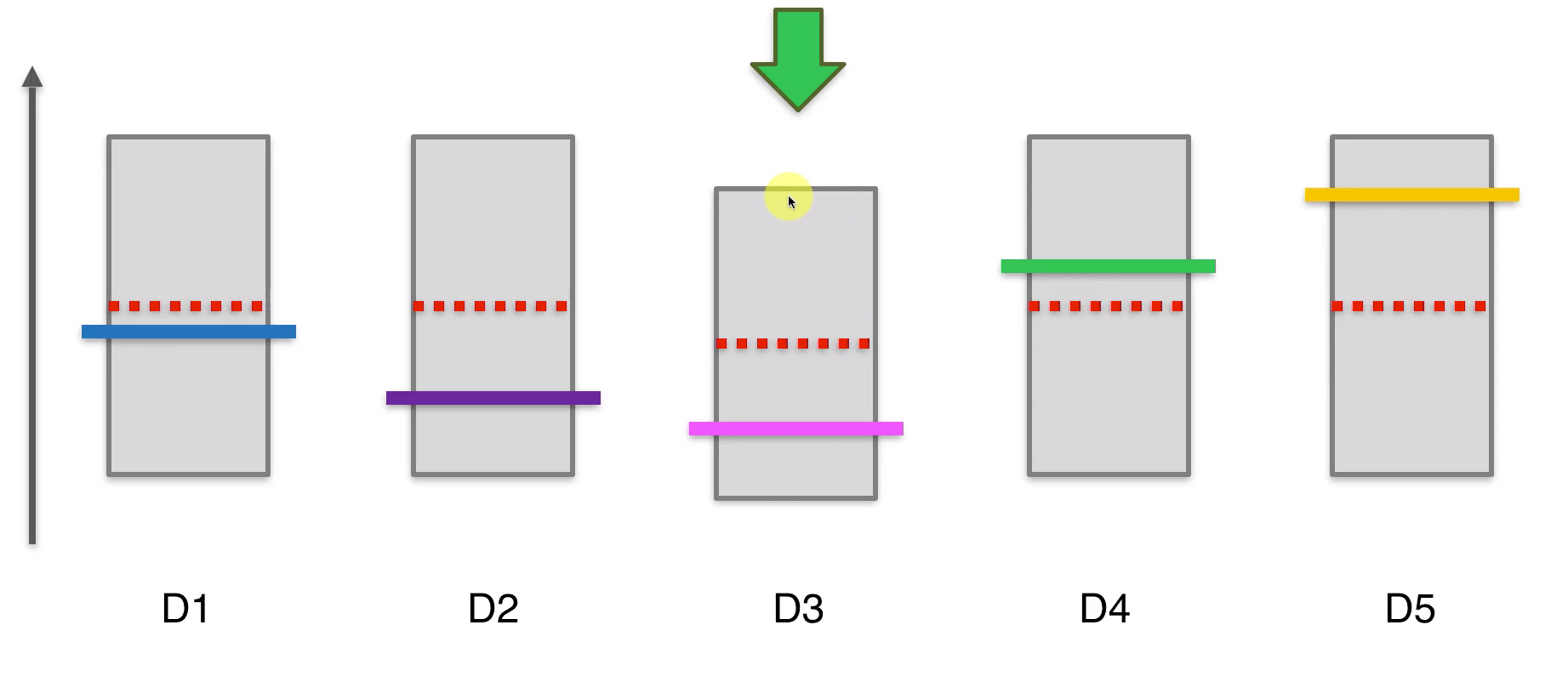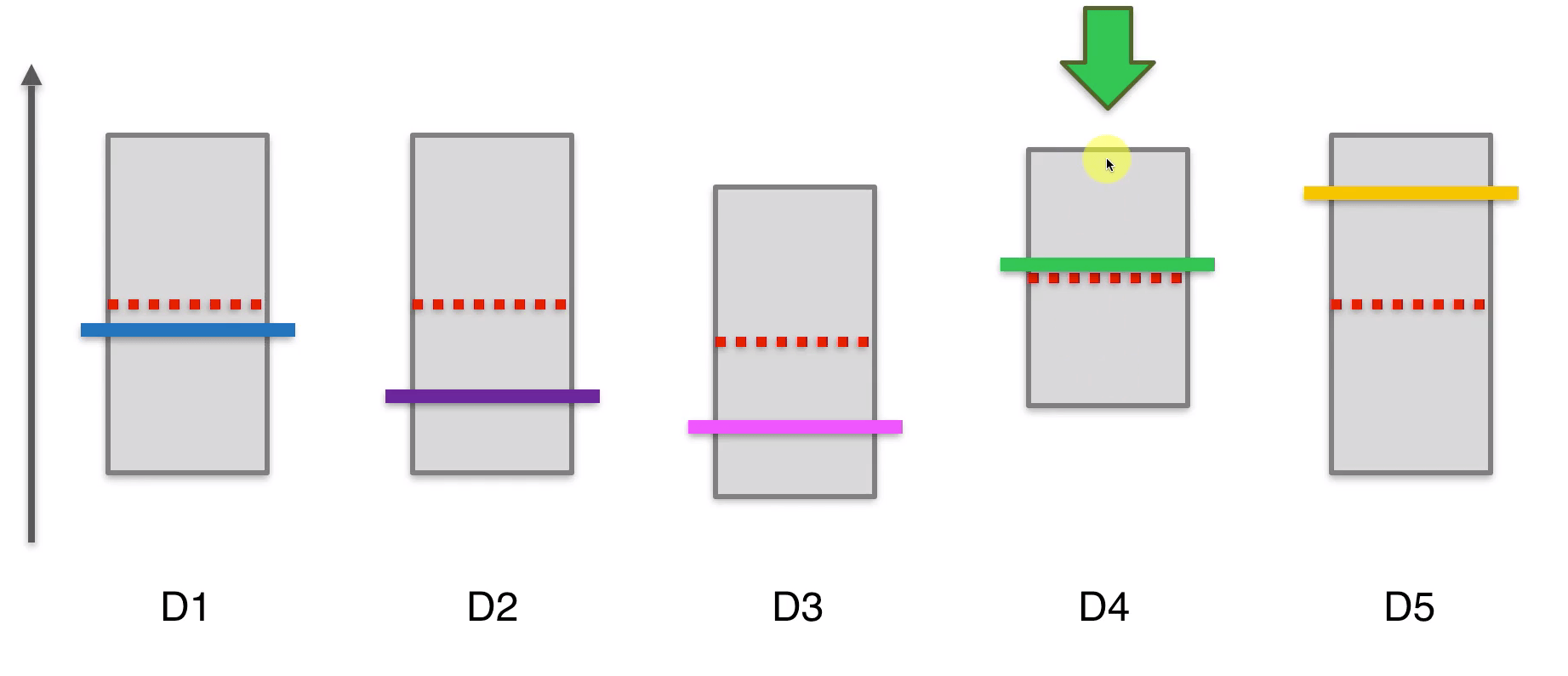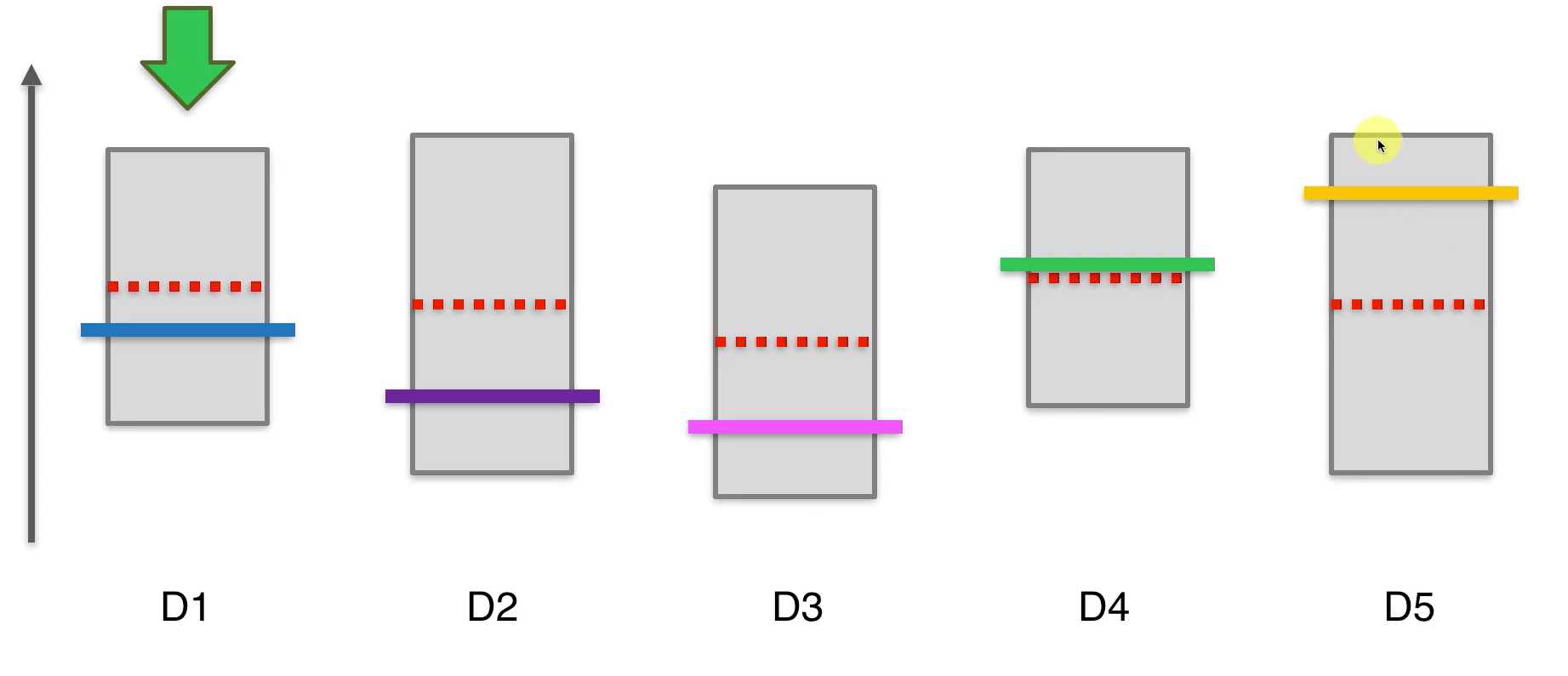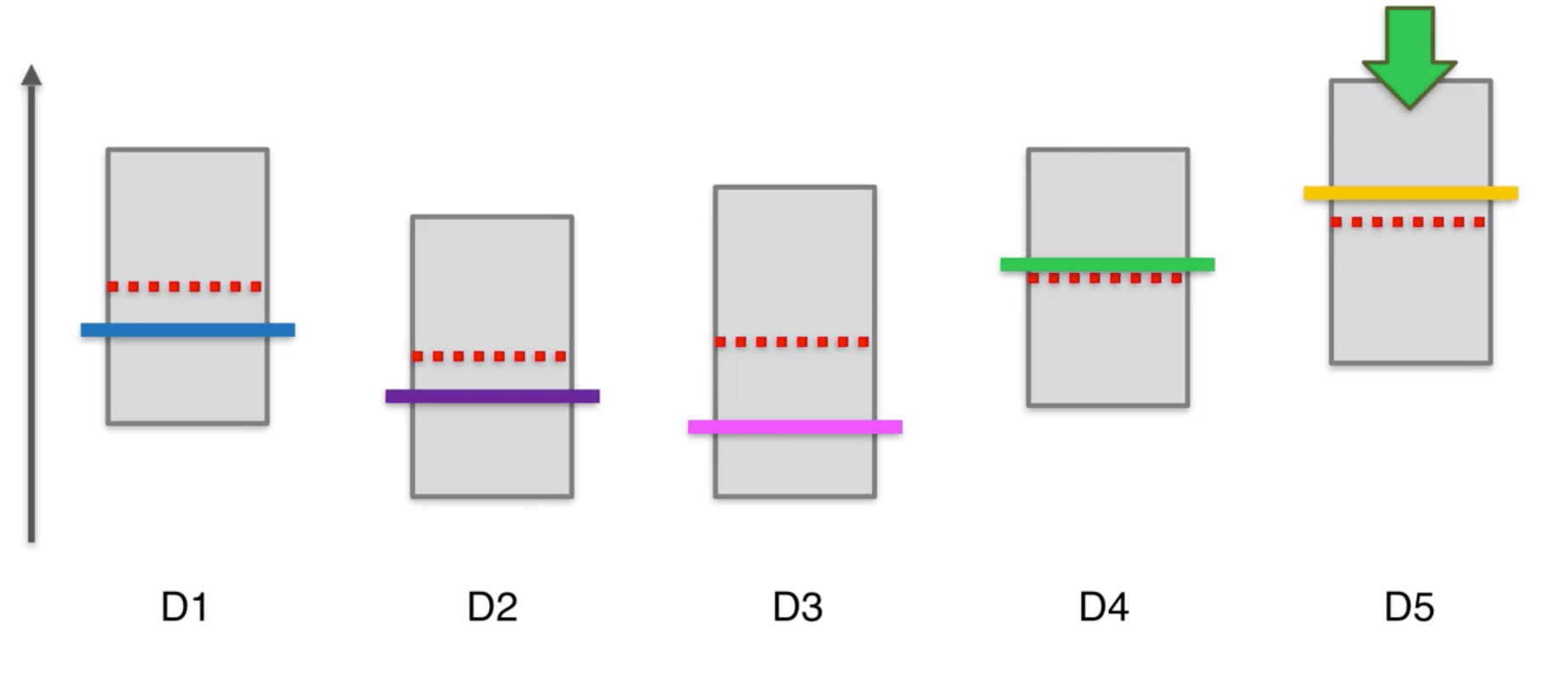UCB算法有一个特点，当我们选择了一个机器很多次后，他的置信区间会变得很小，要给其他的机器一些机会，看看其他机器显示的观察结果所对应的新的置信区间上界是否会更高。这样经过很多轮后，最终D5的选择次数依然会很多很多，他的置信区间会越来越扁，一直到最终轮。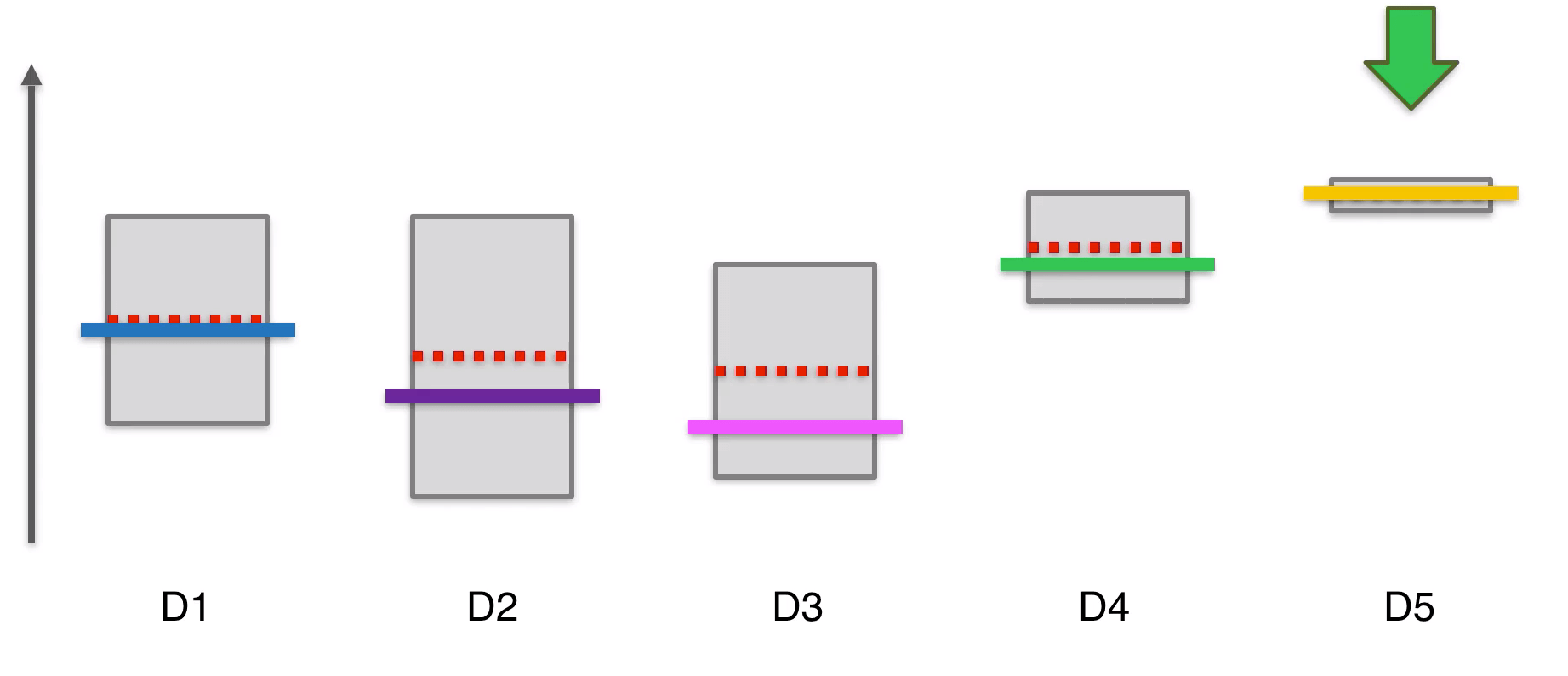## 代码实现

# Importing the libraries
import matplotlib.pyplot as plt
import pandas as pd

# Importing the dataset

# Implementing Random Selection
import random
N = 10000
d = 10
total_reward = 0
for n in range(0, N):
total_reward = total_reward + reward

# Visualising the results
plt.ylabel('Number of times each ad was selected')
plt.show()import matplotlib.pyplot as plt
import pandas as pd
import math

# import the dataset

# Implementing UCB
N = 10000
d = 10
numbers_of_selections =  * d
sums_of_rewards =  * d
total_reward = 0
for n in range(0, N):
max_upper_bound = 0
for i in range(0, d):
if numbers_of_selections[i] > 0:
average_reward = sums_of_rewards[i] / numbers_of_selections[i]
delta_i = math.sqrt(3/2 * math.log(n + 1) / numbers_of_selections[i])
upper_bound = average_reward + delta_i
else:
upper_bound = 1e400
if upper_bound > max_upper_bound:
max_upper_bound = upper_bound
total_reward = total_reward + reward

# Visualising the results